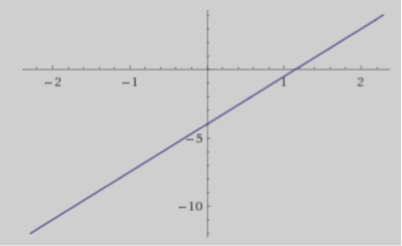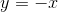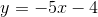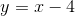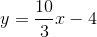## Example Questions

← Previous 1

### Example Question #62 : Coordinate Geometry

What line goes through the points (1, 3) and (3, 6)?

4x – 5y = 4

–2x + 2y = 3

2x – 3y = 5

–3x + 2y = 3

3x + 5y = 2

–3x + 2y = 3

Explanation:

If P1(1, 3) and P2(3, 6), then calculate the slope by m = rise/run = (y2 – y1)/(x2 – x1) = 3/2

Use the slope and one point to calculate the intercept using y = mx + b

Then convert the slope-intercept form into standard form.

### Example Question #4 : How To Find The Equation Of A Line

What is the slope-intercept form of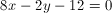?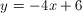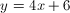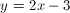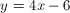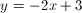Explanation:

The slope intercept form states that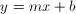. In order to convert the equation to the slope intercept form, isolate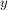on the left side: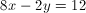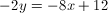### Example Question #5 : How To Find The Equation Of A Line

A line is defined by the following equation: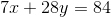What is the slope of that line?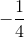Explanation:

The equation of a line is

y=mx + b where m is the slope

Rearrange the equation to match this:

7x + 28y = 84

28y = -7x + 84

y = -(7/28)x + 84/28

y = -(1/4)x + 3

m = -1/4

### Example Question #1 : Lines

If the coordinates (3, 14) and (5, 15) are on the same line, what is the equation of the line?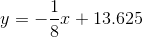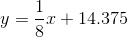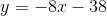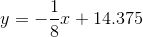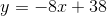Explanation:

First solve for the slope of the line, m using y=mx+b

m = (y2 – y1) / (x2 – x1)

= (15  14) / (5 3)

= (1 )/( 8)

=1/8

y = (1/8)x + b

Now, choose one of the coordinates and solve for b:

14 = (1/8)3 + b

14 = 3/8 + b

b = 14 + (3/8)

b = 14.375

y = (1/8)x + 14.375

### Example Question #31 : Other Lines

What is the equation of a line that passes through coordinates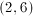and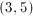?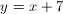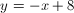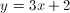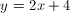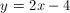Explanation:

Our first step will be to determing the slope of the line that connects the given points.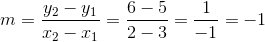Our slope will be. Using slope-intercept form, our equation will be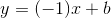. Use one of the give points in this equation to solve for the y-intercept. We will use.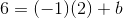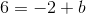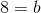Now that we know the y-intercept, we can plug it back into the slope-intercept formula with the slope that we found earlier.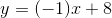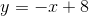### Example Question #32 : Other Lines

Which of the following equations does NOT represent a line?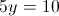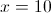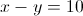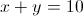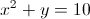Explanation:

The answer is.

A line can only be represented in the form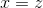or, for appropriate constants,, and. A graph must have an equation that can be put into one of these forms to be a line.represents a parabola, not a line. Lines will never contain anterm.

### Example Question #41 : Other Lines

Let y = 3x – 6.

At what point does the line above intersect the following: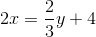(–5,6)

They intersect at all points

(–3,–3)

They do not intersect

(0,–1)

They intersect at all points

Explanation:

If we rearrange the second equation it is the same as the first equation. They are the same line.

### Example Question #1 : How To Find The Equation Of A Line

Which of the following is the equation of a line between the points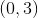and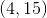?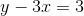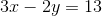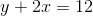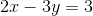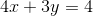Explanation:

Since you have y-intercept, this is very easy.  You merely need to find the slope.  Then you can use the formto find one version of the line.

The slope is: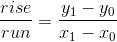Thus, for the pointsand, it is: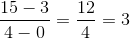Thus, one form of our line is: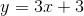If you move theto the left side, you get:, which is one of your options.

### Example Question #2 : How To Find The Equation Of A Line

What is an equation of the line going through points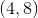and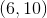?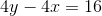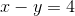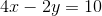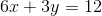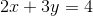Explanation:

If you have two points, you can always use the point-slope form of a line to find your equation.  Recall that this is: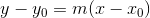You first need to find the slope, though.  Recall that this is:For the pointsand, it is: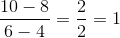Thus, you can write the equation using either point: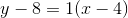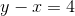Now, notice that one of the options is:This is merely a multiple of the equation we found, so it is fine!

### Example Question #1 : How To Find The Equation Of A Line

Given the graph of the line below, find the equation of the line.